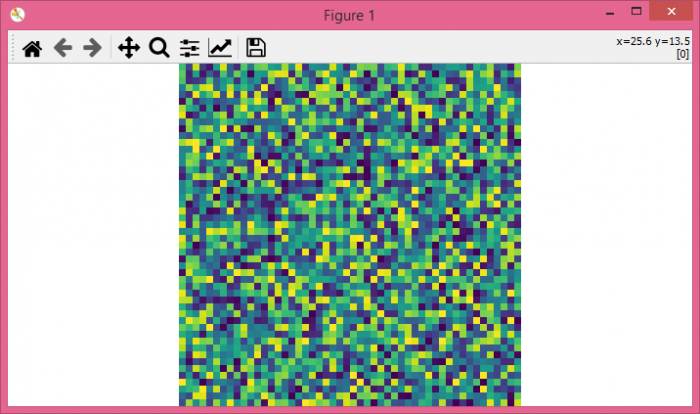# Remove white border when using subplot and imshow in Python Matplotlib

To remove white border when using subplot and imshow(), we can take the following steps −

• Set the figure size and adjust the padding between and around the subplots.
• Create random data points using numpy.
• Get the size of the data.
• Set the figure sizes in inches.
• Get the axes instance that contains most of the figure element.
• Turn off the axes.
• Add axes to the figure.
• Display the data as an image, i.e., on a 2D regular raster.
• To display the figure, use show() method.

## Example

import numpy as np
import matplotlib.pyplot as plt

plt.rcParams["figure.figsize"] = [7.00, 3.50]
plt.rcParams["figure.autolayout"] = True

data = np.random.randint(0, 50, (50, 50))
sizes = np.shape(data)

fig = plt.figure()
fig.set_size_inches(1. * sizes / sizes, 1, forward=False)

ax = plt.Axes(fig, [0., 0., 1., 1.])
ax.set_axis_off()

plt.show()